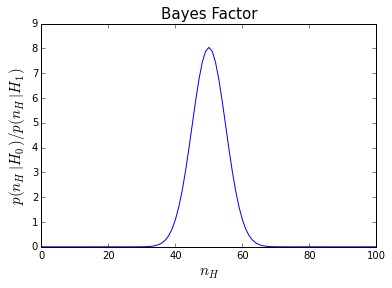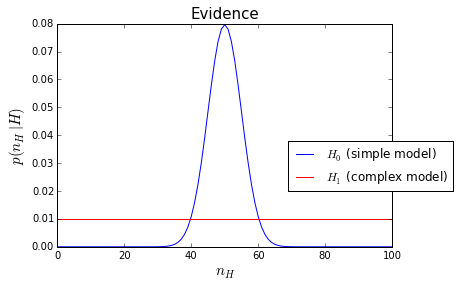# Model Complexity: coin tossing¶

In :
import numpy as np
from scipy.stats import binom
import math
import matplotlib.pyplot as plt
from __future__ import division


## Is the coin fair?¶

Let's imagine flipping a coin $N=100$ times and the data we observe is $n_H=60$ heads. Is the coin fair or biased?

1. First, we will answer this question using the traditional Frequentist Approach by calculating the p-value, i.e. the probability of observing a result at least this extreme give the null hypothesis $H_0$ that coin is fair $$\rm{p-value}=\sum_{n_H \leq 40,\ n_H \geq 60} p(\rm{data}|\rm{fair\ coin})$$

In :
N=100
n=60

# sf is the survival function, i.e. complementary cdf
# ccdf multiplied by 2 because we're doing a two-sided test
print("p-value: ", 2*binom.sf(n, N, 0.5))

('p-value: ', 0.035200200217704813)


The p-value falls below the magic value of 0.05 and so a frequentist would reject the null hypothesis and say that the coin is biased.

2. Now let's try a Bayesian model comparison approach.

What we really want to evaluate is the probability of the hypothesis given the data.

$$p(H|\rm{data})=\frac{p(\rm{data}|H)p(H)}{p(\rm{data})} \ \ \ (\rm{Bayes\ Theorem})$$

There are 2 hypotheses (models), specified by the range of values they take for the coin bias $w$:

• fair coin ($H_0:w=1/2$). Simple model with no free paramters
• biased coin ($H_1:0 \leq w \leq 1$). Complex model with one free parameter
$$\frac{p(H_0|\rm{data})}{p(H_1|\rm{data})} = \frac{p(\rm{data}|H_0)}{p(\rm{data}|H_1)} \frac{p(H_0)}{p(H_1)}$$

Unless we have good reason to believe otherwise, we can take the priors to be equal, $p(H_0)=p(H_1)$. The ratio of evidences on the right-hand side is known as the Bayes factor

$$\rm{Bayes\ Factor}=\frac{p(\rm{data}|H_0)}{p(\rm{data}|H_1)} \\ p(\rm{data}|H_0)=\frac{N!}{(N-n_H)!n_H!} \left( \frac{1}{2} \right)^N \\ p(\rm{data}|H_1)=\frac{N!}{(N-n_H)!n_H!} \int_0^1 w^{n_H} (1-w)^{N-n_H} dw =\frac{1}{N+1}$$

The integral above is a beta function: $$B(a,b) \equiv \int_0^1 w^{a-1} (1-w)^{b-1} dw = \frac{(a-1)! (b-1)!}{(a+b-1)!} \rm{\ (when\ a\ and\ b\ is\ a\ positive\ integer)}$$

Therefore the Bayes factor for the coin problem is $$\rightarrow \rm{Bayes\ Factor}=\frac{(N+1)!}{n!(N-n)!2^N}$$

In :
def BF(n,N): # Bayes Factor for coin problem
h0=(1/2)**N
h1=math.factorial(n)*math.factorial(N-n)/math.factorial(N+1)
return h0/h1

In :
print "Bayes Factor ",BF(60,100)

Bayes Factor  1.09523053788


The data appears to very slightly favour the simpler hypothesis (!), the opposite conclusion of the frequentist approach. This is an example of how Bayesian methods naturally invoke Occam's razor (law of parsimony).

In :
x=np.arange(0,N+1,1)
y=[BF(i,N) for i in x]
plt.plot(x,y)
plt.title("Bayes Factor",fontsize=15)
plt.xlabel("$n_H$",fontsize=15)
plt.ylabel("$p(n_H|H_0)/p(n_H|H_1)$",fontsize=15)
plt.show()In :
def EH0(n): # evidence for the H_0 hypothesis, i.e. p(data|H_0)
return math.factorial(N)/(math.factorial(n)*math.factorial(N-n))*((1/2)**N)

In :
x=np.arange(0,N+1,1)
y=[EH0(i) for i in x]
plt.plot(x,y) # plotting p(data|H_0)
plt.plot([0,N],[1/(N+1),1/(N+1)],'r') # plotting p(data|H_1)
plt.legend(('$H_0$ (simple model)','$H_1$ (complex model)'),bbox_to_anchor=(1.2,0.5))
plt.title('Evidence',fontsize=15)
plt.ylabel('$p(n_H|H)$',fontsize=15)
plt.xlabel('$n_H$',fontsize=15)
plt.show()In this figure, we see Occam's principle in action. The more complex model can predict a more extreme range of data, but in situations where the data can be explained by both models (near $n_H=50$) the evidence favours the simpler model.